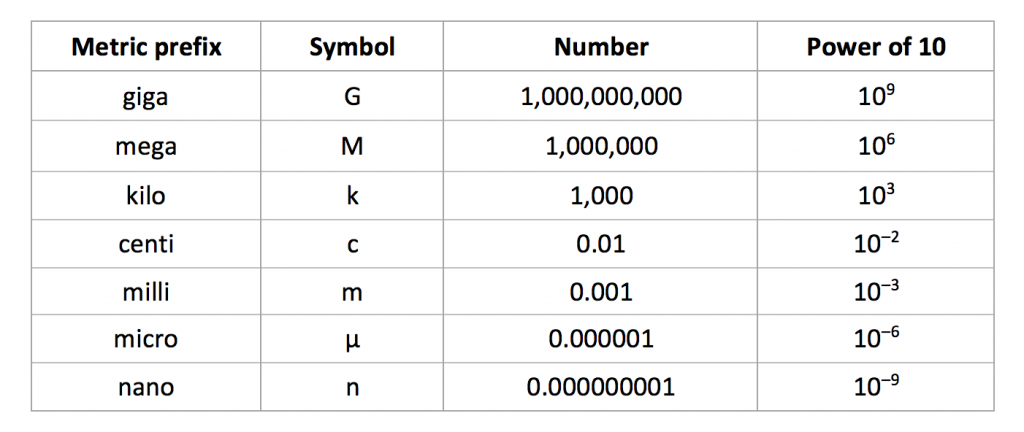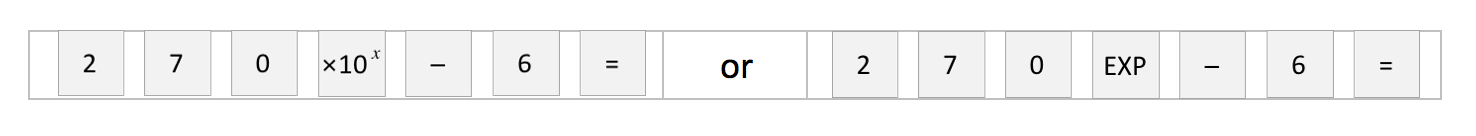Physics 14/01/2019

# Maths skills in GCSE Physics

By KEVIN QUINN

In this blog, we look in detail at number-handling techniques you need to know for the GCSE exams, including standard form and metric prefixes.

## Reminder

Before you read this blog, remind yourself of the maths skills discussed in four earlier blogs:

Make sure you know and understand the information in these blogs (and this one!) before the exams.

## Standard form

Physics is the subject of everything, from the very small (such as atoms) to the absolutely enormous (such as galaxies). The problem is that writing down really small or really large numbers can be tricky, and it’s easy to make mistakes. For this reason, we often use standard form in physics.

To write a number in standard form, we just need to make it look like this:

A × 10B

where A is a number between 1 and 10 ( to be precise) and B is a positive or negative whole number.

EXAMPLE 1.  Write 300,000,000 m/s in standard form.

SOLUTION.  Work out how many places the decimal point has to be moved to produce a number between 1 and 10. If we move the decimal point (which is at the end of the number) 8 places to the left, we end up with 3.

We then have to multiply this by 10 to the power of the number of places moved, which gets us to the final answer of 3 × 10m/s. Another way of doing this question is to first write 300,000,000 as 3 × 100,000,000.  Because 100,000,000 = 108, we can then write our final answer as 3 × 10m/s.

EXAMPLE 2.  Write 0.00072 s in standard form.

SOLUTION. To write 0.00072 in standard form, we have to work out how many places the decimal point has to be moved to produce a number between 1 and 10.

Here, we need to move the decimal point 4 places to the right to end up with 7.2. The fact that we’ve moved it 4 places to the right means that we have to multiply 7.2 by 10–4  to end up with the correct answer of 7.2 × 10–4 s.

From the above examples, you can see that:

• When the number is GREATER than 10, to write it in standard form the 10 in our final answer will be raised to the power of a POSITIVE whole number,
• When the number is LESS than 1, the 10 in our final answer will be raised to the power of a NEGATIVE whole number.

## Metric prefixes

We’ve just seen how standard form allows us to write really large or small numbers in a brief, easy-to-understand way. Metric prefixes provide us with a different way of doing the same thing. A metric prefix is a symbol which goes before a unit to tell us how big or how small it is. The prefixes you need to know for your GCSE exams are listed below:EXAMPLE 1.  Convert 2.5 GJ (2.5 gigajoules) into joules.

SOLUTION.  Here, all we have to do is multiply 2.5 by 10(or 1,000,000,000) to give an answer of 2.5 × 10(2,500,000,000) J.

EXAMPLE 2.  Convert 400 nm (400 nanometres) into metres.

SOLUTION.  This one is a little trickier. As ‘nano’ means 10–9, we multiply 400 by 10–9 (or 0.000000001) to give an answer of 4 × 10–7 (0.0000004) m.

## Using a calculator for standard form

Your scientific calculator has a standard form button. Knowing how to use it will save you time in the exam, time which will allow you more time for other questions.

For example, if you wanted to include 270 μm (converted to metres) in a calculation, rather than punching in 270 × 10–6 in its entirety, you could (depending on the calculator you have) enter either:Kevin Quinn